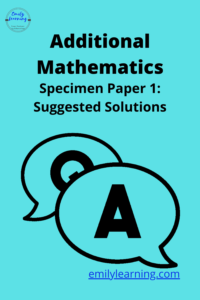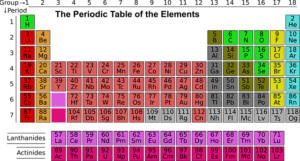# BlogHere, you will find the suggested solutions to the O Level Add Math Specimen Paper 2. The specimen paper can be downloaded from the Singapore…Here, you will find the suggested solutions to Add Math Specimen Paper 1. The specimen paper can be downloaded from the Singapore Examinations and Assessment…## Format of O Level Additional Mathematics (syllabus 4049)

The Singapore O Level Additional Mathematics (also called A- Math or Add- Math) consists of 2 papers. Any of the topics in the Add Math…## What’s tested in O Level Additional Mathematics (syllabus 4049)

Do you know what’s tested in O Level Additional Mathematics? Well, the easiest way to find out is to look at your textbook. The latest…## Adapting to solve this almost linear simultaneous equation for O Level Add Math

Simultaneous equations are often a part of a question in O level Additional Math (also called A Math or Add Maths in short). You can…## Solving Simultaneous Equations – O Level Additional Mathematics

Get our simultaneous equations free course based on the Singapore additional Math syllabus right now! This course consists of 3 video lectures and 1 worksheet…## Periodic Table for O Level Chemistry

Periodic Table O- Level Chemistry is what I call a buffer topic in O Level Chemistry. It is a relatively easy-to-understand topic and there isn’t…